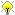[Documentation] [TitleIndex] [WordIndex]Please ask about problems and questions regarding this tutorial on answers.ros.org. Don't forget to include in your question the link to this page, the versions of your OS & ROS, and also add appropriate tags.

# Angles

Description: Convenience class for abstractly storing and representing angles.

Keywords: ecl angles

Tutorial Level: BEGINNER

## Overview

The angle class is a convenience class that automates storage and angle wrapping for you. It stores the angle in radians (which any good engineer/mathematician should default to!). Take this into consideration if using it where speed is of the essence. That is, don't use Angle and then do all your calculations in degrees - there will be alot of copying and converting.

## Basic Usage

Note that you can templatise the storage as either float or double. Using float is sometimes important for low end embedded boards which have to do alot of angle calculations.

```   1 #include <ecl/geometry/angle.hpp>
2
3 using ecl::Angle;
4 using ecl::wrap_angle;
5
6 // ...
7
8 Angle<double> a(3.33); // automatically wraps on -pi:pi
9 double degrees = a.degrees();
10 Angle<double> b = a + 3.24; // again, will automatically wrap the answer.
11 // RotationMatrix is currently a typedef for a 2d eigen matrix
12 Angle<double>::RotationMatrix matrix = a.rotationMatrix();
```

## Functions

For those who just wish to use functions rather than objects:

```   1 // wrapping
2 double angle = wrap_angle(3.33);
3
4 // conversions